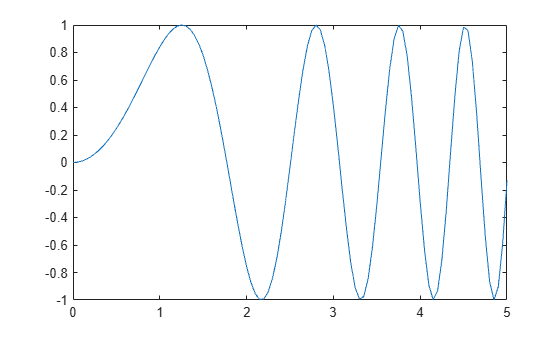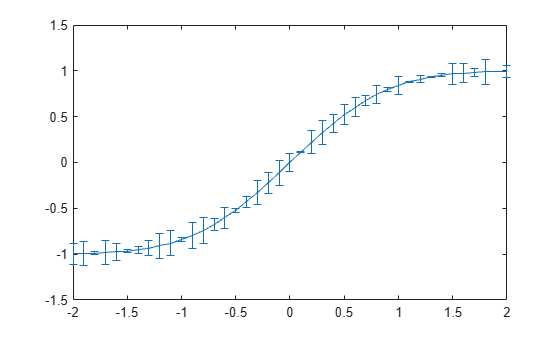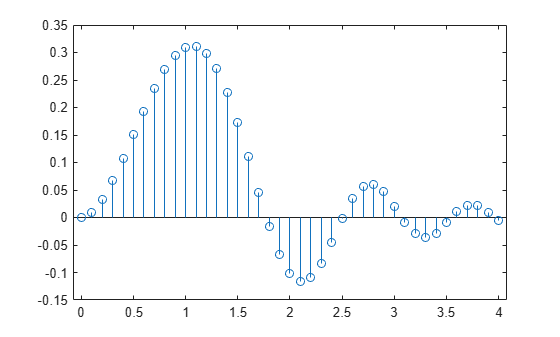Main Content

# Create Common 2-D Plots

This example shows how to create a variety of 2-D plots in MATLAB®.

### Line Plots

The `plot` function creates simple line plots of x and y values.

```x = 0:0.05:5; y = sin(x.^2); figure plot(x,y)```Line plots can display multiple sets of x and y data.

```y1 = sin(x.^2); y2 = cos(x.^2); plot(x,y1,x,y2)```### Bar Plots

The `bar` function creates vertical bar charts. The `barh` function creates horizontal bar charts.

```x = -2.9:0.2:2.9; y = exp(-x.*x); bar(x,y)```### Stairstep Plots

The `stairs` function creates a stairstep plot. It can create a stairstep plot of Y values only or a stairstep plot of x and y values.

```x = 0:0.25:10; y = sin(x); stairs(x,y)```### Errorbar Plots

The `errorbar` function draws a line plot of x and y values and superimposes a vertical error bar on each observation. To specify the size of the error bar, pass an additional input argument to the `errorbar` function.

```x = -2:0.1:2; y = erf(x); eb = rand(size(x))/7; errorbar(x,y,eb)```### Polar Plots

The `polarplot` function draws a polar plot of the angle values in `theta` (in radians) versus the radius values in `rho`.

```theta = 0:0.01:2*pi; % angle rho = abs(sin(2*theta).*cos(2*theta)); % radius polarplot(theta,rho)```### Stem Plots

The `stem` function draws a marker for each x and y value with a vertical line connected to a common baseline.

```x = 0:0.1:4; y = sin(x.^2).*exp(-x); stem(x,y)```### Scatter Plots

The `scatter` function draws a scatter plot of x and y values.

```load patients Height Weight Systolic % load data scatter(Height,Weight) % scatter plot of Weight vs. Height xlabel('Height') ylabel('Weight')```Use optional arguments to the `scatter` function to specify the marker size and color. Use the `colorbar` function to show the color scale on the current axes.

```scatter(Height,Weight,20,Systolic) % color is systolic blood pressure xlabel('Height') ylabel('Weight') colorbar```Download ebook